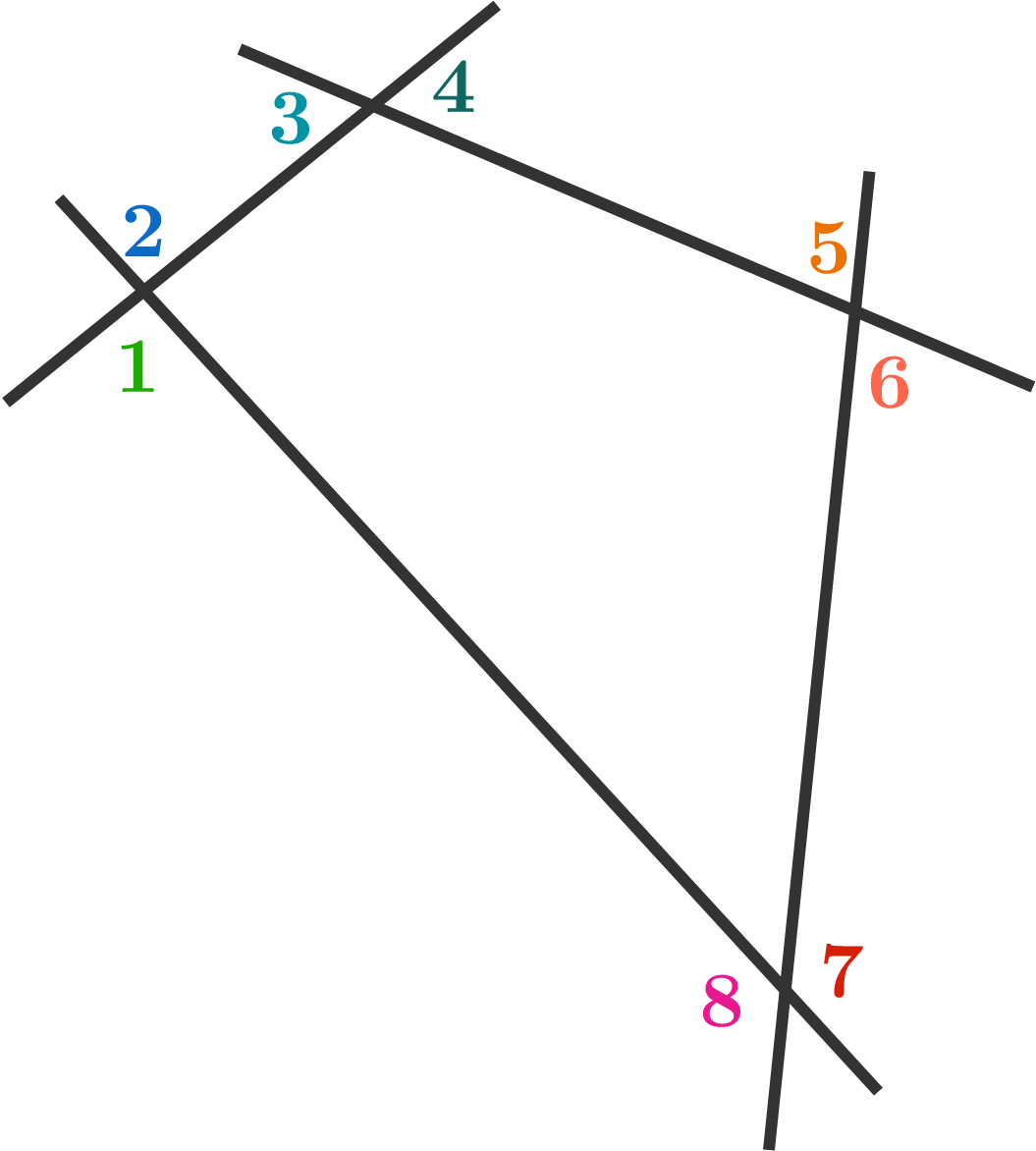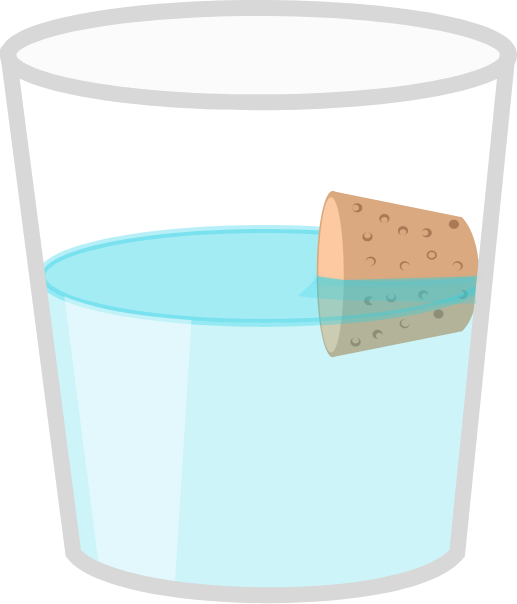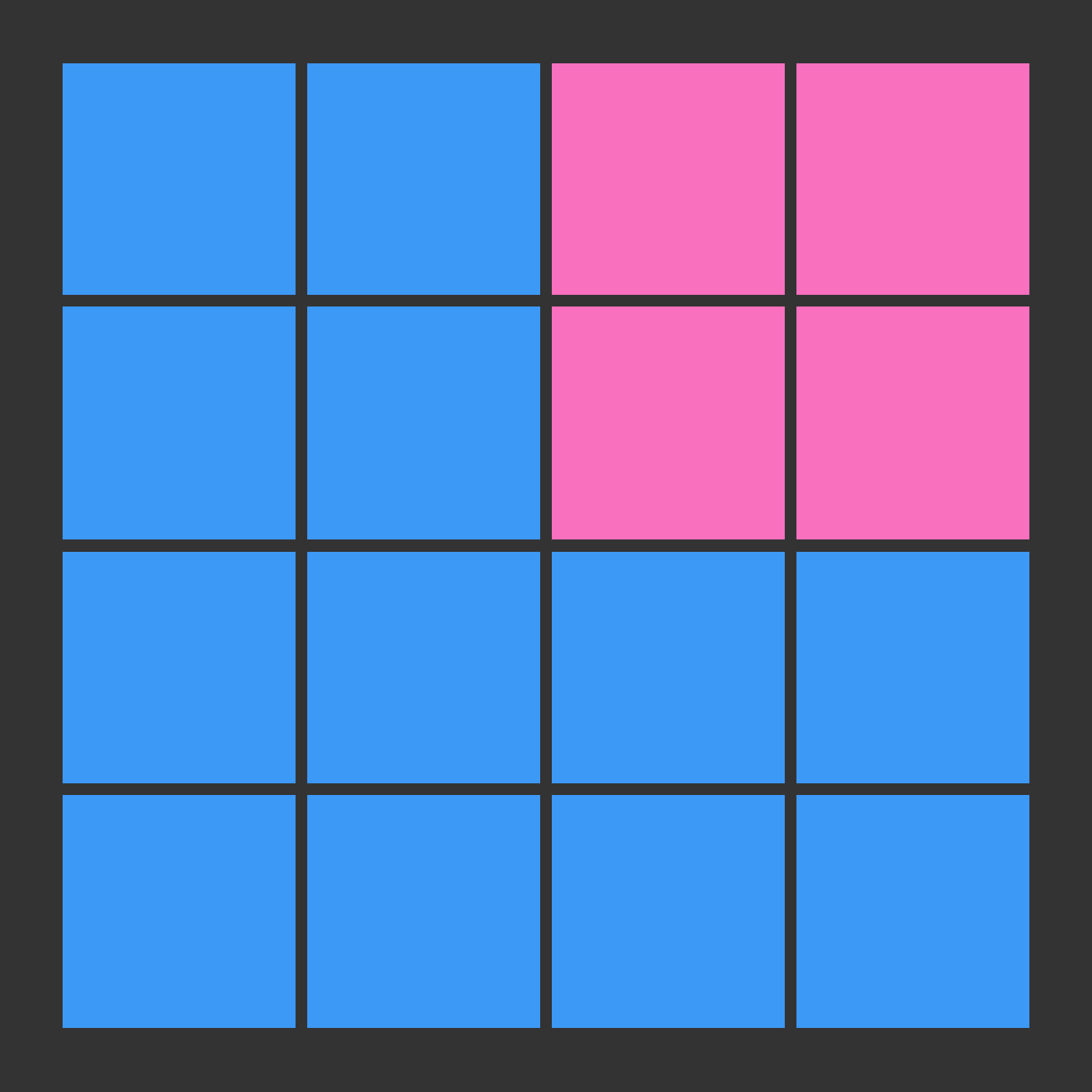# Problems of the Week

Contribute a problem

# 2017-09-25 Basic

True or False?

The sum of two positive irrational numbers must be irrational.

What is the sum of the angles numbered 1-8?If you place a cork in a half-filled glass of water, it will always drift to the side of the glass.

Is there a way to get the cork to float in the horizontal center of the glass?

If so, how can this be accomplished? If not, why not?

A biologist wants to encode a large genetic sequence consisting of the letters C, G, A, and T.

In his data, C and G were the most common letters, so he chose the encoding C -> 1, G -> 0, A -> 00, T -> 01. For example, CCGT is encoded as 11001.

Later, the biologist noticed 11001 in his codebase. He concluded that it represented CCGT. Is he correct?

The following code allows you encode a DNA string. Try running the code, and then change the string in line 12 to see if you can find other strings with the same encoding!

encoding = {'C' : '1', 'G' : '0', 'A' : '00', 'T': '01'}

def encode(sequence):
#Create an empty string for the encoding of the sequence
encoded_string = ""

#For each letter, append its encoding to the encoded string
for letter in range(len(sequence)):
encoded_string += encoding[sequence[letter]]
return encoded_string

print(encode('CCGT'))
Python 3
You need to be connected to run code

Bonus 1: Can you describe a better encoding scheme?

Bonus 2: Can you write code that returns all possible genetic sequences from a given encoding?Example of an arrangement that follows the rule.

In a $4 \times 4$ grid, each cell is colored either pink or blue such that each cell shares an edge with at least two cells of the same color.

Is it necessarily true that there is a $2 \times 2$ square in the grid of one color?

×